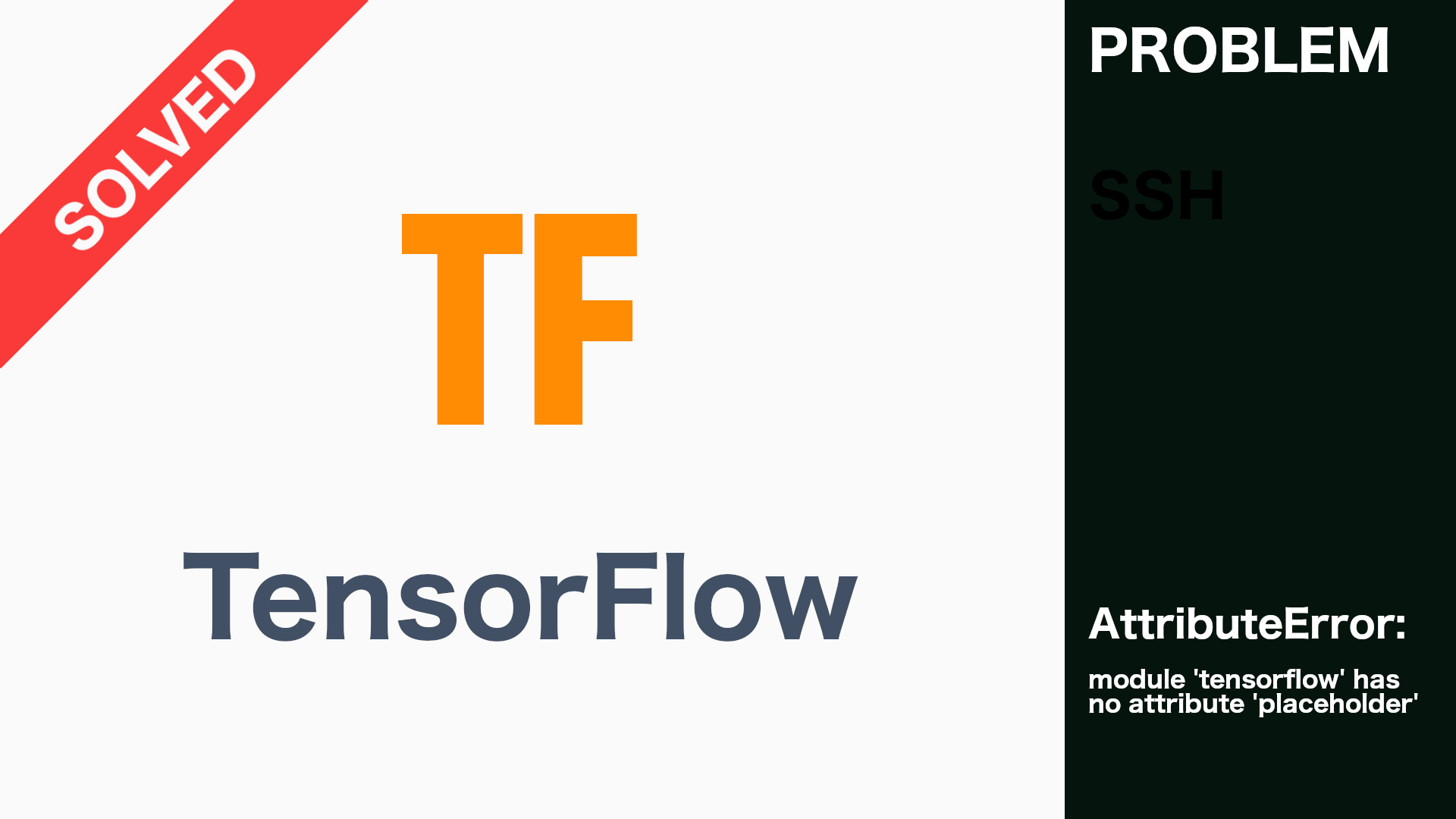## [SOLVED] – AttributeError: module ‘tensorflow’ has no attribute ‘placeholder’

The cause of the mentioned problem is incompatibile code with installed tensorflow library. In this case you have code compatible with tensorflow 1.0 version but installed tensorflow 2.0 or higher. Let’s see what you can do to solve the problem.

## Solution 1. Follow tensorflow migration guide

Migrate your code following this guide. Solution for the title problem is to use variables instead of placeholders. Let’s see the following example:

```import tensorflow as tf

x = tf.placeholder(shape=[None, 2], dtype=tf.float32)```

## Solution 2. Use tensorflow 1.x compatibility mode

The second approach is to use tensorflow v1 copatiblity mode. To dot his you have to use import tensorflow.compat.v1 as tf instead of import tensorflow as tf and add tf.disable_v2_behavior().

```import tensorflow as tf

x = tf.placeholder(shape=[None, 2], dtype=tf.float32)```
```import tensorflow.compat.v1 as tf

tf.disable_v2_behavior()
x = tf.placeholder(shape=[None, 2], dtype=tf.float32)```

That’s all what I’ve prepared for you in this tutorial, if I helped you, please consider sharing this post to help me gain a wider audience.
Thanks and I hope to see you in my next tutorial.